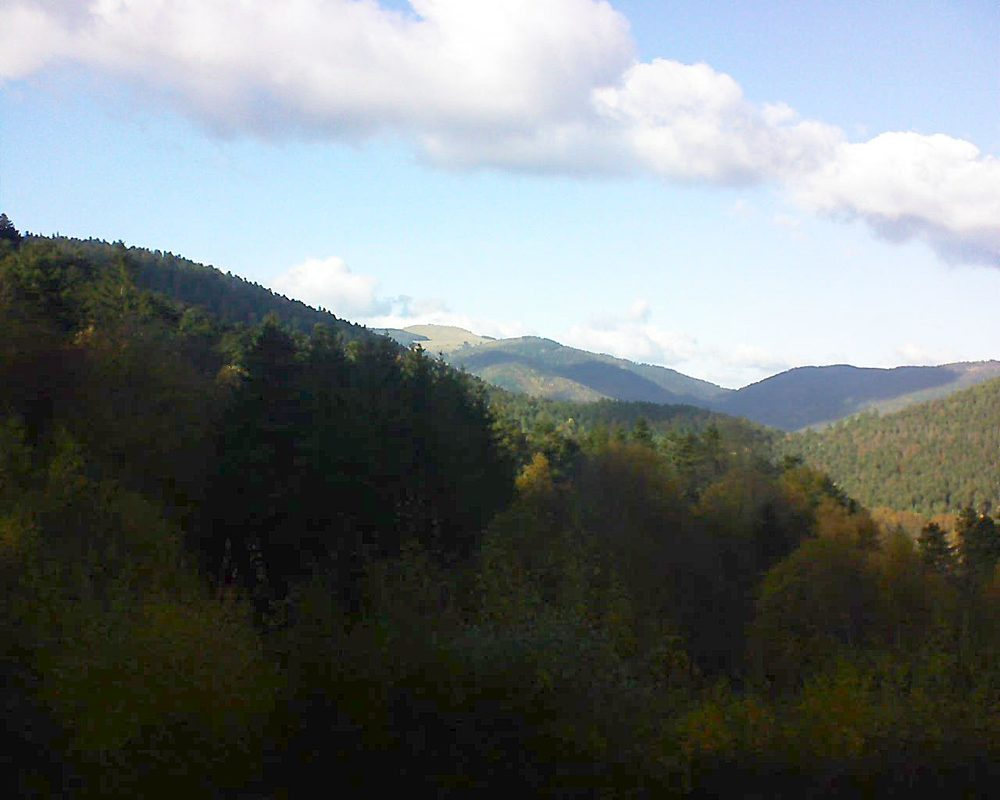Le petit ballon (Alsace, Vosges)
vu depuis l'Hôtel Restaurant Domaine Langmatt-1M  -14j  -7j  -3j  -1j  -3h  -1h  -15m   08.11.2021 14:30   +15m  +1h  +3h  +1j  +3j  +7j  +14j  +1M

Webmasters, insérez cette image actualisée toutes les 15 minutes sur votre site :
http://webcam.langmatt.net/image.jpg

The The
right-hand left-hand
sentence contains sentence contains
four a's, one b, three c's, four a's, one b, three c's,
three d's, thirty-nine e's, three d's, thirty-five e's,
ten f's, one g, eight h's, seven f's,four g's,eleven h's,
eight i's, one j, one k, eleven i's, one j, one k,
four l's,one m,twenty-three n's one l, one m, twenty-six n's
fifteen o's, one p, one q, fifteen o's, one p, one q,
nine r's, twenty-three s's, ten r's, twenty-three s's,
twenty-one t's, four u's, twenty-two t's, four u's,
seven v's, six w's, three v's, five w's,
two x's, five y's, two x's, five y's,
and one and one
z. z.

-- Sallows, Lee